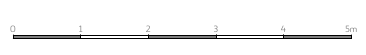# What is scale? Learn the definition

## Definition

Let's start by getting to know the definition of the scale

1. scale, in geometry a real number equal to the ratio of the distance of the images of the points to the distance their counterimages in some geometric projections, such as monocurrency, rectangular affinity and others A special case is the cartographic scale , which expresses the ratio of the distance measured on the map to distances on the Earth's surface in projections that are approximately similar (ie in mapping small areas of the Earth's surface to a plane)
2. scale fixed ratio of decrease or increase of the drawing, map, model in comparison with the size of the real object

The word scale may be used in other senses and may be used in other fields. Our Scale Calculator is only for to calculate scales related to distances (lengths).

### Map scale

There are three ways to write the scale in geography

1. Numerical scale - this is an entry in the form 1 : 1000, which should be read as: one in a thousand. It means that the object presented on the map (or the project) is reduced by 1000 times.
2. The nominated scale - an example of such a notation is: 1 cm - 1 km. Means one centimeter on the map corresponds to one kilometer in reality.
3. Linear graduation - this is a graphical representation of the scale:From the example above, we know that the entire section on the map corresponds to five meters in reality.

### Model scale

In modeling, scale is used to indicate how many times a given model has been reduced in relation to its actual dimensions. Most often it is written as a fraction, e.g. 1/24 (i.e. 1:24)

In the case of modeling, many scales are so popular that you can, for example, build a collection of airplanes on this scale, which will look great on the shelf.

In railway modeling, scales are also specified with letters and e.g. the Z scale means 1/220, TT 1/120 a H0 1/87 scale.

### The scale of the project

Project is a set of technical drawings showing how the facility is to be built. Such drawings are made on a certain scale, e.g. 1: 100, 1: 200. On its basis, we can find out the real dimensions of the object, to be built.